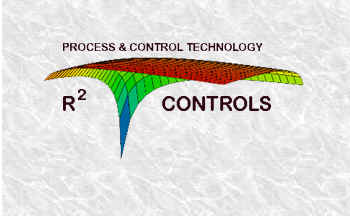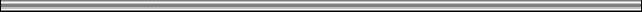Welcome to R2 Controls### MATHCAD 3-D avi

Who says you can't display 4 dimensions on a CRT display.  R2 Controls has done lot's of modeling.  In order to explain how a model works X-Y type graphs can be very helpful.  Even simple models can involve several variables and to see how they are related 3-D graphs literally add a new dimension to a simple X-Y plot.  Excel is not so good for making 3-D graphs unless you have a graphing "add in" like AXUM.  MathCAD however provides an additional degree of freedom by allowing the animation of a worksheet.  When applied to a 3-D graph, the results can be quite revealing if the models can output the data in a suitable form.  Imagine a tablecloth flowing over the edge of a falling object and you get the picture.  MathCAD and AXUM are available from http://www.mathsoft.com.

• AVI #1 is R2 Controls Logo but animated - This is a model of the gross profit in \$/Day for a distillation column at a gas plant in Louisiana.  The X-Axis is the iso-butane in the n-butane product, the Y-axis is the n-butane in the more valuable iso-butane product.  The Z-axis is profit.  The downward spike in the graph of R2 Controls logo is a result of enormous energy costs as the products approach perfect purity.

Surface of increasing energy cost.avi

• AVI #2 is using the distillation column model as above but instead of varying the energy cost we vary the relative value of the products.  iso-butane sell price is gradually increased as the time axis.  This definitely shifts the optimum operating point.

Surface of increasing iso value.avi

• AVI #3 is the exact same surface as shown above except we animate a contour plot rather than a surface plot.  In many ways the contour plot is more useful to see how the optimum operating point shifts with increasing value of the iso-butane product.

Contour of increasing iso value.avi

• AVI #4 Comes from a compressor project.  As gas well pressure declines it takes ever more Compressor power to recover a given quantity of Gas.  Imagine an Electric drive Compressor sucking gas out of a well.  The compressor power is expensive and limits how much gas can be recovered.  As well pressure drops more electric power is required to recover the gas.  Pick a price for electric power and you can see how the optimal gas recovery drops as the well pressure declines (over a period of years).

Optimization Contour of declining well pressure.avi

Note: these *.AVI files are about 1 MB each and may take a while to load if you have a slow connection.  You will also need a mediaplayer.  You can download Windows Media Player from http://microsoft.com